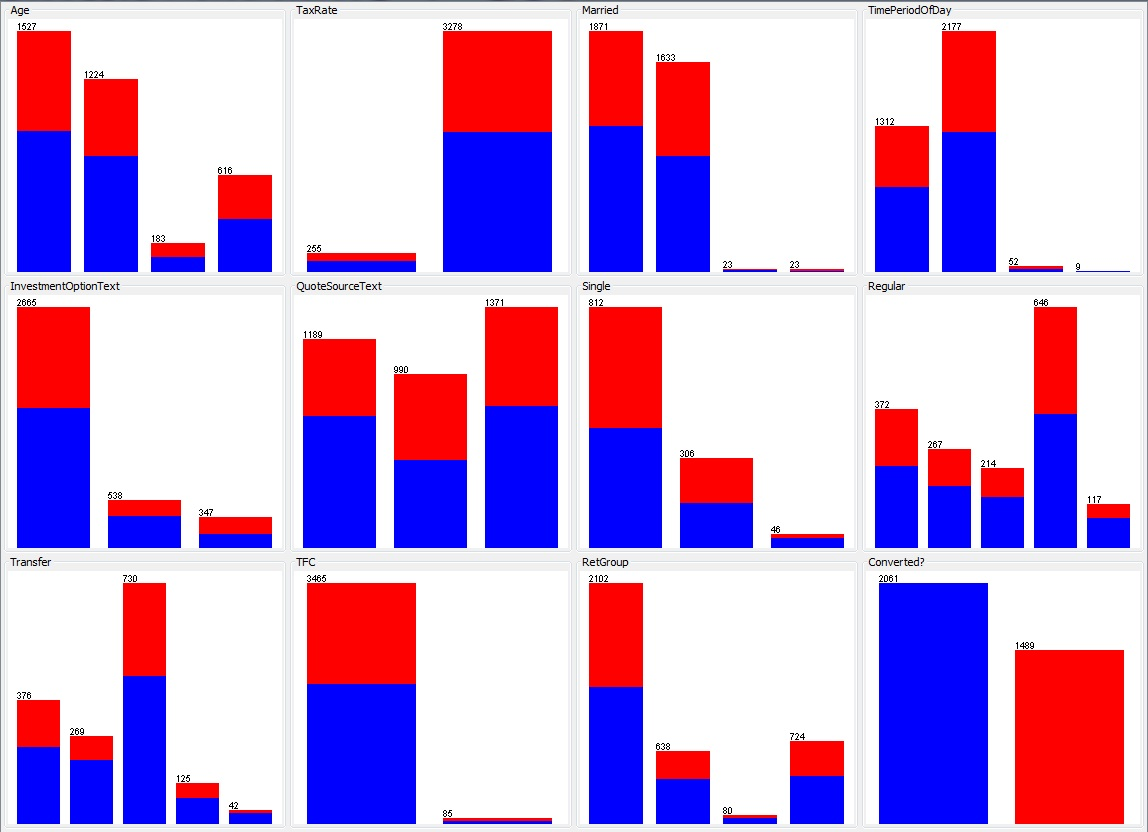# Prediction: not all that easy?

Predicting if a potential sale is converted into an actual sale, based on a information about the potential sale, is a classification problem. We are trying to classify each potential sale into a ‘converted’ or ‘not converted’ class. However its not always going to be possible. The following set of graphs show Weka’s pre-processing parameters plot:The final graph (bottom right) shows the outcome from the training data that has been captured: blue indicates ‘not converted’ and red indicates ‘converted’, the ratio is approximately 40% converted and 60% not The preceding eleven graphs show various parameters (e.g. customer’s age category or product variant); each category within the graph is a stacked bar showing the converted and non-converted ratio for that subset. Visually it can be observed that the ratios of converted to non-converted are fairly consistent across all the parameters; there are a few exceptions but these are all in the much smaller subsets. This is not a promising start, could a combination of two parameters be the answer? Weka allows us to visualise each pair of parameters with a plot matrix:If any combination of two parameters were a good classifier then we would start to see asymmetrical distributions of the colours in the plots at the intersection of those parameters. Note the top and right rows are the parameter being predicted which is why the colours clearly separate into a row or column – effectively these are what was shown in the previous group of charts.

Maybe three or more parameters would produce a good classifier; to achieve this visually some more dimensions would be needed but this is not going to work beyond at most 3. Fortunately there are numerous classification algorithms, many of which have implementations in Weka, which will handle multiple parameters. Unfortunately for the dataset in question no classifiers produced a reasonable result  so it would appear the parameters selected (because they were readily available) do not have a measurable influence on outcome.